Capitol College

Spring Semester 2000

# Circuit Theory: EE - 159

Lab Experiment #2

## Series and Parallel Circuits

Andy Buettner

Instructor: Dr. Thomas

Due: February 18th, 2000

Received: ___________________Objectives

The Objectives of this lab were to study the relationships that exist within series - parallel circuits, and to be able to calculate and measure current through specific points. Additionally, use that knowledge to indirectly calculate the internal resistance of a power source.Equipment Used

1. Equipment used

1. ET - 3100 Trainer ser no: 1614

2. Fluke DMM ser no: 31101515

1. Components used

1) EE - 159 lab kit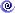Procedures

1. ### Part 1: Series Circuits

1. Assemble Circuit and record individual resistances

2. Power the circuit to 5 volts

3. Measure the voltage across each resistor

4. Calculate currents through each resistor

5. Measure the current through the whole circuit

1. Part 2: Parallel Circuits

1. Assemble the circuit and measure the resistance of each resistor, and the total resistance of the circuit

2. Power the circuit to 5 volts and record the currents through each resistor

3. Calculate the current through each resistor

1. Part 3: Internal Resistance

1. Set the trainer to exactly 1.5v

2. Connect a 100-ohm resistor between the positive and negative terminals.

3. Measure the voltage, and calculate the internal voltage drop and resistance.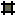Schematic Diagram for part III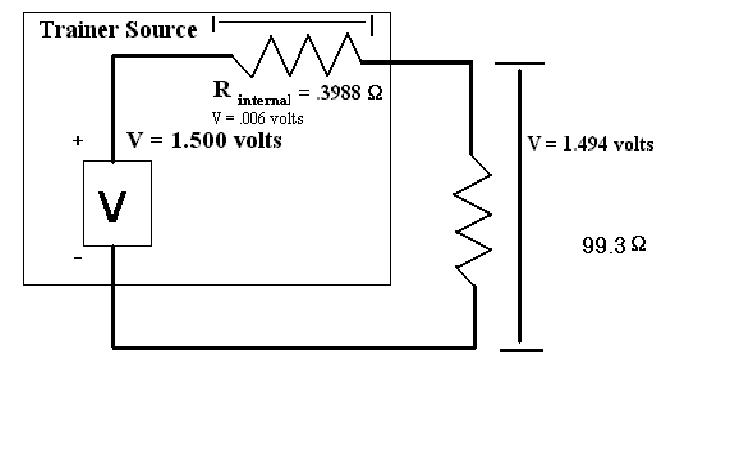Results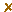Table #1: Part 1 master

 Resistor 1 Resistor 2 Resistor 3 Measured Resistance 151.6W 329.5W 459.0W Measured Voltage .804V 1.754V 2.443V Calculated Current 5.303ma 5.323ma 5.322ma Measured Current 5.27ma 5.27ma 5.27ma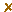Table #2: Part 2 master

 Resistor #1 Resistor #2 Resistor #3 Measured Resistance 1.195KW 2.197KW 3.248KW Measured Voltage 5.00V 5.00V 5.00V Calculated Current 4.184ma 2.295ma 1.539ma Measured Current 3.846ma 2.187ma 1.488maAnswers to Questions

1. Q: Compare the calculated currents with the obtained in Table 1: Is the difference expected and why?

A: Yes, the internal resistance of the DMM caused the difference.

1. Q: Do the same for table 2.

A: Yes, the internal resistance caused a voltage drop across the circuit thus causing a decrease in current.

1. Q: What was the internal resistance of the Trainer?

A: 3.988W

1. Q: What is the percent voltage regulation of the Trainer?

A: 0.40%

1. Q: Is Rtotal equivalent to what is expected?

A: Yes.

1. Q: Is the total voltage drop in part 1 equal to 5 volts?

A: The total calculated voltage drop is 5.001V; therefore, yes.

1. Q: Was the Rtotal what was expected?

A: Yes.

1. Q: Does each current added up equal Itotal?

A: Itotal = 8.018ma: Yes, they are equal.

1. V2 = 4 volts

2. V2 = 4.0 volts

3. V2 = 3.87 volts: The meter creating a significant difference in the total resistance causes the difference.

4. V2 = 3 voltsConclusions

My experiences have shown that current is easier and more accurate to derive than any other value in the Ohm's law equation. Additionally, making current measurements adds the most error to results than measuring any other value. Also, calculating the internal resistance of the Trainer had a lot of error in it as the only digit that would have value would be the last digit, which had little significance. A different source, or a better way of determining the voltage drop would be needed.Attachments

1. Original handouts

2. Original data

3. Calculations